# Generating Reflectance Curves from sRGB Triplets (Page 3)

#### Linear Least Squares (LLS) Method

Since there are so many more columns of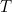than rows, the linear system is under-determined and gives rise to an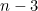dimensional subspace (33-dimensional in our case) of reflectance curves for a single sRGB triplet. There are well-established techniques for solving under-determined linear systems. The most common method goes by various names: the linear least squares method, the pseudo-inverse, the least-squares inverse, the Moore-Penrose inverse, and even the “Fundamental Color Space” fundamental metamer.

Suppose we pose this optimization problem: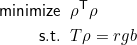This linearly constrained minimization can be solved easily by forming the Lagrangian function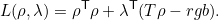The solution can be found by finding a stationary point of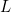, i.e., setting partial derivatives with respect to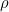and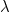equal to zero: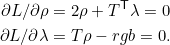Solving this system by eliminatinggives the LLS solution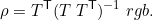Thus, a reflectance curve can be found from a sRGB triplet by simply converting it to linear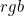and multiplying it by a 3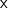3 matrix,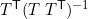. Unfortunately, the resulting solution is sometimes not very useful. Consider its application to this reflectance curve, which represents a bright red object color: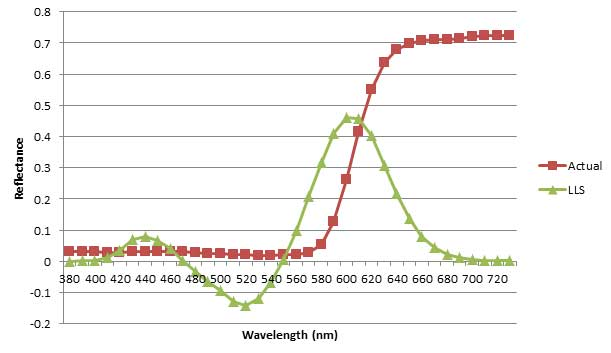Reflectance curve for Munsell 5R 4/14 color sample and linear least-squares reconstruction.

The LLS solution contains negative reflectance values, which don’t have physical meaning and limit its usefulness in realistic applications. Computationally, this is a very efficient method. The matrixcan be computed in advance, as shown here, and each new sRGB value needs only be multiplied by it to get a reflectance curve.